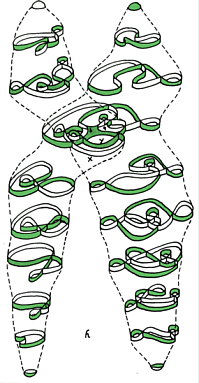Tuesday,  March 29

More on Surfaces
Adventures on the Mobius Band, the Klein Bottle, and
the Projective Plane !

"New" Surfaces and
The Classification of Surfaces

What about graphs on the Torus?
We have a connected graph on the torus with one vertex, two edges and only one region. But this information does not match for the euler formula for the plane or the sphere. SO.. the torus must be topologically different from the sphere or the plane!
In fact for the torus we see that it is possible for V+R= E   or  V-E+R= 0

We learned about (and proved) Euler's Formula for the plane or the sphere. What can we say about a formula for the torus or the Klein bottle?

For any connected graph in the plane or on the sphere, V+R = E + 2.

Surfaces:Mark Sudduth's web page of surfaces.[ A physics master's degree student at UT, Arlington.]

What is a surface?
Bounded, unbounded:
Closed, open:

With or without boundary:
Orientable or Non-orientable:
Consider the moebius band and the Klein Bottle as examples of non-orientable surfaces.
Can be realized (imbedded) in a plane, in 3 space, in 4 space.
Can be visualized (immersed) in ...

Examples:A closed disc, an open disc, a plane, an annulus- cylinder, a mobius band;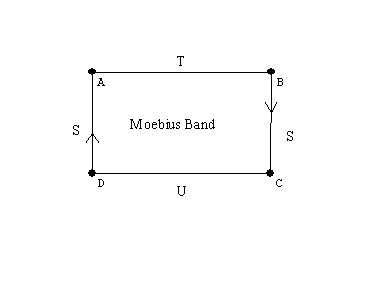Experiments with the mobius band:
Draw a curve along the center of the band- we cover both "sides."
Cut along that curve- the band does not fall apart, but gets twice as long!

a sphere

a torus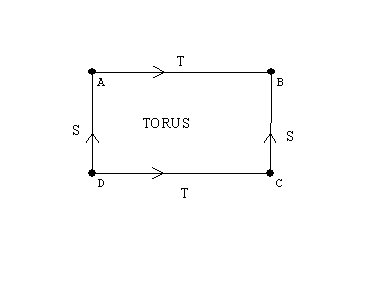a Klein bottle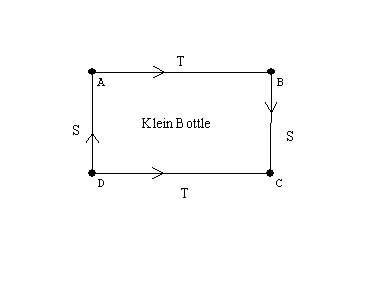# A javaview visualization of the Klein bottle,

the projective plane... Why is this a closed and bounded surface?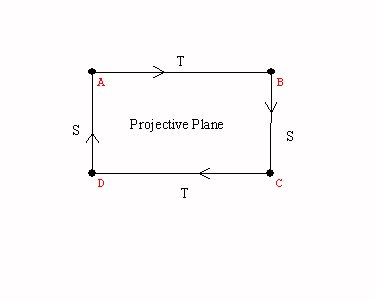A sphere with a cross cap.

Review Activity:Graphs on the torus. [redone as homework for this class]
Games and puzzles on the torus and the klein bottle.

" If Mathematicians Made Pretzels" Proof without words.
The following two figures are topologically equivalent.

From the Fun Fact files, hosted by the Harvey Mudd College Math DepartmentFigure 1Figure 2

Imagine that the two objects in Figure 1 are solid (with thickness) and made of very flexible and stretchy rubber.

Question: is it possible to deform one object into the other in a continuous motion (without tearing or cutting)?

Hint: it is important that the object is solid and has thickness; this transformation cannot be done with a one-dimensional piece of string. It is also not possible to do this with a piece of rope because even though the rope has thickness, it is not flexible or "stretchy" enough. See below for an explanation and animated gif. Or, don't scroll down if you want to think about it a while!

The Math Behind the Fact:
One way to do this is the following. Widen one of the loops and move one of its handles along the stem between the two loops to the other loop and push it through the hole so that the two loops become unlinked. The reference contains a sequence of pictures of this transformation.

Graeme McRae has generously contributed the animated gif in Figure 2, showing another solution to this problem! (Thank you, Graeme!)
Here is the original picture: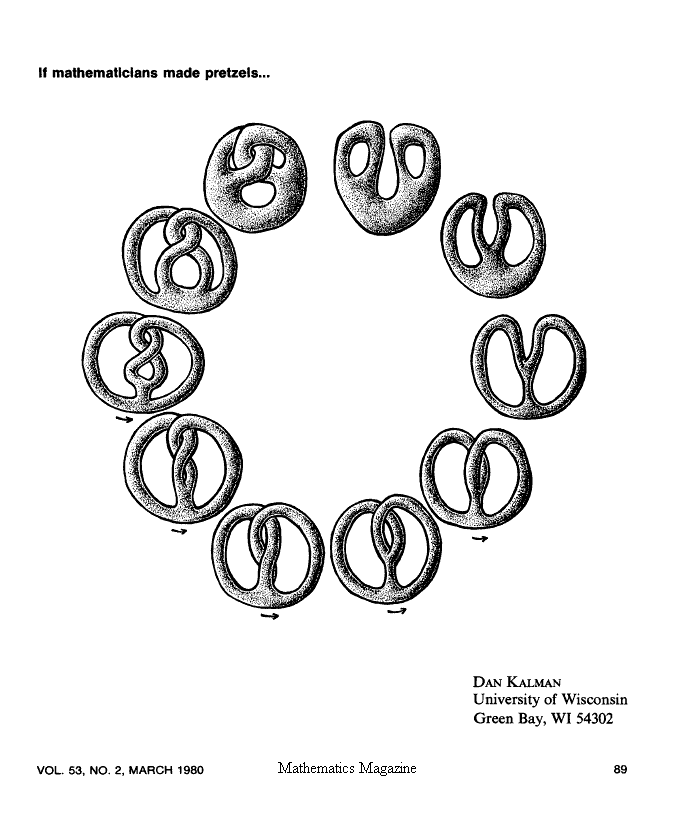Ways to think of surfaces : cross-sections/ projections/moving curves/ using color to see another dimension.   ChromaDepthTM 3D;    CD Image gallery
How do 3d glasses work? |
Spheres with handles,

Visualizations of surfaces by flattened - cut apart models.
A cylinder, a mobius band, the torus, the Klein bottle, the projective plane.

Closed Surfaces: Handles and cross-caps  attached to the sphere.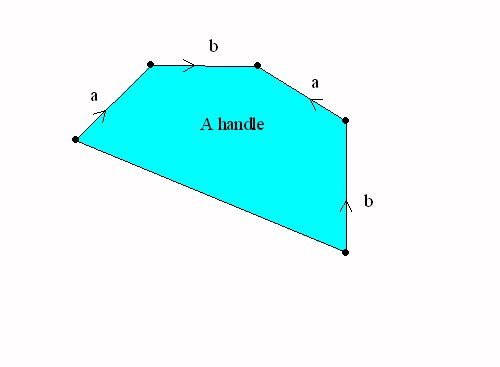A sphere with a handle = a torusA Sphere with a cross cap = the projective plane

The Topological Classification of "closed surfaces."

Every connected closed and bounded surface is topologically equivalent to a sphere with handles and crosscaps attached.

Post proof remarks:
This classification determines the euler characteristic of each surface.
If the surface is orientable, it is a sphere with n handles, so V-E+R = 1 - 2n +1 = 2-2n.  For example the torus has euler characteristic 2-2*1=0.
If the surface is non-orientable, then  is it a sphere with k crosscaps and n handles, so the euler characteristic is V-E+R = 1 - (k+2n) +1 =2 -2n -k.
Notice that a sphere with two cross caps has euler characteristic 0, the same as the torus. But this was the euler characteristic of the Klein Bottle. So we should be able to recognize the Klein bottle as a sphere with two cross caps.
This can be done by a single normalization of one pair of edges with the same  orientation.

Other interest in surfaces: Examples
Ways to think of surfaces : cross-sections/ projections/moving curves/ using color to see another dimension.

Generalization of surfaces are called "manifolds".  cross sections / projections/ moving surfaces-solids.
"minimal surfaces" (FAPP video?)
Transforming surfaces: "turning the sphere inside out." video